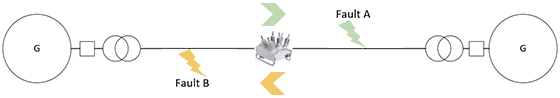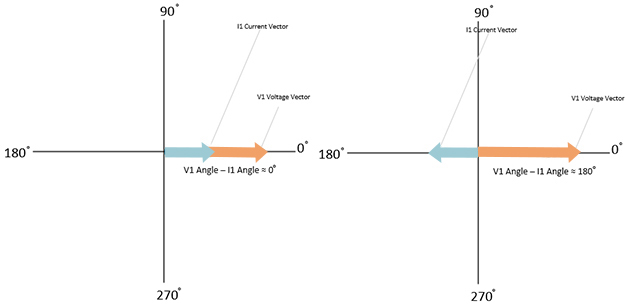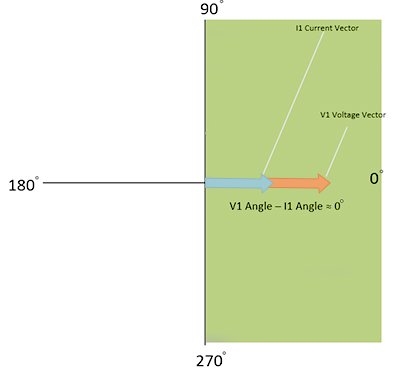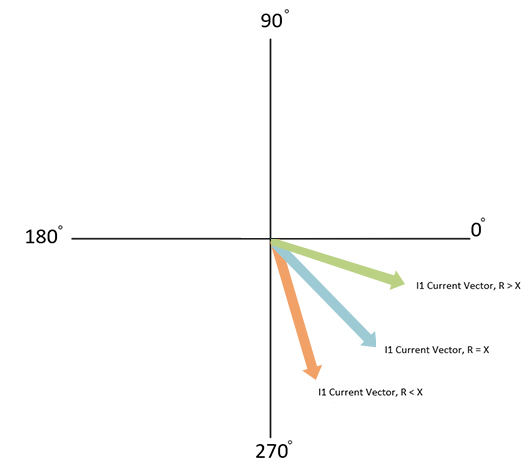Press Release June 2018

# Concept Primer: Directional Overcurrent

Explaining the underlying physics of Directional Protection

Distributed Generation around the world is an emerging technological trend, which poses a new set of challenges for the energy industry. With the real possibility of bi-directional power flow and the erosion of the conventional Generation, Transmission, Distribution, Retail sequence, the inevitable outcome is that distribution networks need to handle network protection in both forward and reverse power flow scenarios.

While directional protection is not a new concept, it provides a significant degree of additional complexity in comparison to traditional magnitude based protection schemes. This mini-series of technical review articles will cover the underlying physics concepts to help engineers and technicians understand the concepts behind directional protection and for what applications it is most suited.

“Distribution networks with a high penetration of solar renewable energy connections often run backwards during the day (power flow towards the substation) and forwards at night (power flow away from the substation),” reports NOJA Power Group Managing Director Neil O’Sullivan. “This phenomenon is becoming increasingly common and requires Directional Protection implemented on all protection devices installed on these feeders to provide correct fault detection and protection co-ordination.”

## Directional Overcurrent

Directional Overcurrent is our first element for examination. Conceptually, this element provides the most simple understanding for calculation, outcomes and testing, but it is certainly useful to understand the principles behind how it operates in the field.

Overcurrent is typically applied for faults which don’t include an earth leakage. This class of faults include lines clashing together (a phase to phase fault), a branch falling across the lines, or some short circuit connection between phases. It is not the best protection to detect earth faults.

Philosophically, Directional Overcurrent is the calculation of the difference between the phase angle of the current and the phase angle of the voltage

To oversimplify, if the voltage and current vectors point the same way, it's probably forward, and if they point in opposite directions, the fault is probably reverse.

The typical industry practice for relays is to convert the phase measured currents and voltages to sequence components (Positive, Negative and Zero Sequence Voltages and Currents). Overcurrent Direction is calculated on the difference between the angle of the Positive Sequence Voltage and the Positive Sequence Current.

Let’s explore a fault scenario to get this understanding. Consider the following situation:Figure 1 – A Simple Network with Two Sources

We have a source on either side of this network, and depending on the configuration, the fault could either be forward or reverse from the perspective of the midline Recloser (an automatic circuit breaker with integrated Current Transformers, Voltage Sensors and Microcontroller relay).

Firstly, let us take the most simple case, when we assume the cable is a resistor. The Qualitative vectors for the Positive Sequence Voltage and Current are in figures 2 and 3 below.Figure 2 and 3, A forward and Reverse purely resistive fault

From this diagram, we will consider Fault A as a forward fault. The fault angle then between V1 and I1 would be approximately 0 degrees. For the converse case, a reverse fault, the difference would be approximately 180 degrees.

Commonly, the fault angle range is up to 90 degrees either side of the “most forward/reverse case”.

If the Relay Characteristic Angle (RCA) indicated the fault was forward from -90, through 0 to 90 degrees, the fault would be picked up as forward, and reverse for anything else.Figure 4: the Operating Zone for an RCA of 0 Degrees

But why do we care about such a large range of angles for a forward fault?

The reality is that an electricity distribution network is not purely resistive, and the angle shift relationship between Voltage and Current depends on both the reactive and resistive components of the network – the X and R values.

Conceptually, when we look at the fault vector diagram, the resistive values are along the x axis, and the reactive values are along the y axis. The more reactance (which could be capacitive or inductive), the more the vectors move away from the resistive line.

The reason that engineers set an operating range, is to catch all the cases for a forward fault, whilst rejecting all the reverse cases (or vice versa). Depending on your cable choices and network, the amount of X and R depending on the fault distance from the circuit breaker will result in very different angle readings.

 Characteristic 95mm2 Al 240mm2 Al Resistance 0.31 Ω/km 0.12 Ω/km Reactance 0.15 Ω/km 0.3 Ω/km X/R Ratio 0.48 2.5

The table above outlines a few values for an example comparison between two Aluminium Conductors. One is a 95mm2 cross section, and the other a 240mm2. The interesting value here is the X/R ratio. The X/R ratio shows that for a given length of line, you can expect a certain amount of reactance and resistance.

When the ratio is below 1, the longer the cable, the more resistive it is. That is, the further the fault is away from your circuit breaker, the closer the voltage-current vector difference will be to zero.

When the ratio is above 1, the longer the cable, the more reactive it is. The further the fault is away from your circuit breaker, the further the voltage – current vector difference will be apart.

In other words, depending on your cable, the vector difference will either diverge or converge because of the ratio between X and R. Figure 5 shows qualitative current vectors based on the X and R relationship.Figure 5: Different Resultant Currents based on X/R

The reason that Directional overcurrent has a “Relay Characteristic Angle” or “Torque Angle” (a legacy term), is to account for the reactance of your network. You can assume that if the forward angle drifts based on reactance, then a similar drift will happen in the opposite direction. Therefore, a Directional Overcurrent setting of say 60 degrees assumes a fairly reactive network, with resultant operating ranges being from 150 degrees, down through 60 degrees and down to -30 degrees. The reverse cases would be the opposite.

In summary, we explored a few key points:

• Directional Overcurrent is determined through multiple techniques, but the most common is through a comparison between Positive Sequence Voltage angle and Positive Sequence Current Angles.
• If the network was purely resistive, a fair assumption would be that forward has a vector difference of zero, and reverse faults have a vector difference of 180 degrees.
• The biggest reason for phase shift between voltage and current is the X/R ratio at a fault site.
• All practical networks have some reactance, and the reason for a Relay Characteristic Angle is to account for all the Overcurrent Fault Cases, as the ratio between X and R varies along the length of the protected feeder.
• The more resistive a network is, the closer the RCA is to zero degrees. The more reactive it is, the closer the RCA is to -90 degrees.

The NOJA Power OSM Recloser system is deployed in countless directional protection situations around the world. If you need support with configuring your device or would like to learn more, get in contact at www.nojapower.com.au

This content is provided in good faith, and NOJA Power accepts no liability or responsibility for any loss or damages resulting from inaccuracies or omissions in this content. NOJA Power are committed to sharing knowledge and expertise in the energy industry, and would welcome any feedback or corrections regarding this content. Feedback can be directed to NOJA Power at NOJASales@nojapower.com.au